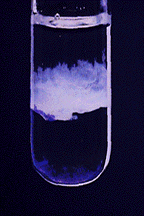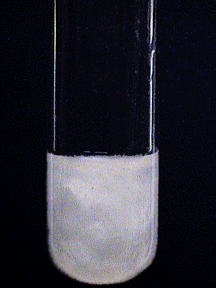# Characteristic Reactions of Magnesium Ions (Mg²⁺)

$$\newcommand{\vecs}{\overset { \rightharpoonup} {\mathbf{#1}} }$$ $$\newcommand{\vecd}{\overset{-\!-\!\rightharpoonup}{\vphantom{a}\smash {#1}}}$$$$\newcommand{\id}{\mathrm{id}}$$ $$\newcommand{\Span}{\mathrm{span}}$$ $$\newcommand{\kernel}{\mathrm{null}\,}$$ $$\newcommand{\range}{\mathrm{range}\,}$$ $$\newcommand{\RealPart}{\mathrm{Re}}$$ $$\newcommand{\ImaginaryPart}{\mathrm{Im}}$$ $$\newcommand{\Argument}{\mathrm{Arg}}$$ $$\newcommand{\norm}{\| #1 \|}$$ $$\newcommand{\inner}{\langle #1, #2 \rangle}$$ $$\newcommand{\Span}{\mathrm{span}}$$ $$\newcommand{\id}{\mathrm{id}}$$ $$\newcommand{\Span}{\mathrm{span}}$$ $$\newcommand{\kernel}{\mathrm{null}\,}$$ $$\newcommand{\range}{\mathrm{range}\,}$$ $$\newcommand{\RealPart}{\mathrm{Re}}$$ $$\newcommand{\ImaginaryPart}{\mathrm{Im}}$$ $$\newcommand{\Argument}{\mathrm{Arg}}$$ $$\newcommand{\norm}{\| #1 \|}$$ $$\newcommand{\inner}{\langle #1, #2 \rangle}$$ $$\newcommand{\Span}{\mathrm{span}}$$$$\newcommand{\AA}{\unicode[.8,0]{x212B}}$$

• Most common oxidation state: +2
• M.P. 650º
• B.P. 1120º
• Density 1.74 g/cm3
• Characteristics: Magnesium is a silvery metal that is quite active, reacting slowly with boiling (but not cold) water to give hydrogen and the rather insoluble magnesium hydroxide, $$\ce{Mg(OH)2}$$. It combines easily with oxygen and at high temperatures reacts with such nonmetals as the halogens, sulfur, and even nitrogen.

## Characteristic Reactions of Mg²⁺

Magnesium ion rarely forms complex ions. All salts are white; most are soluble in water.

### Aqueous Ammonia

Aqueous ammonia precipitates white gelatinous $$\ce{Mg(OH)2}$$:

$\ce{Mg^{2+}(aq) + 2NH3(aq) + 2H2O(l) <=> Mg(OH)2(s) + 2NH4^{+}(aq)} \label{eq1}$Ammonium salts dissolve $$\ce{Mg(OH)2}$$ or prevent its precipitation, when added to aqueous ammonia. This is a buffer effect and shifts the pH to a lower value, causing a shift of the precipitation equilibrium in Equation \ref{eq1} to the left.

### Sodium Hydroxide

Sodium hydroxide gives the same precipitate as aqueous ammonia:

$\ce{Mg^{2+}(aq) + 2OH^{-}(aq) <=> Mg(OH)2(s) }$### Sodium Monohydrogen Phosphate

$$\ce{Na2HPO4}$$ gives a characteristic crystalline precipitate in an ammonia-ammonium chloride buffer.

$\ce{ Mg^{2+}(aq) + NH3(aq) + HPO4^{2-}(aq) <=> MgNH4PO4(s) }$### Magnesium Reagent

Solid magnesium hydroxide forms a blue "lake" with a dilute solution of 4-(p-nitrophenylazo)resorcinol (magnesium reagent).### No Reaction

$$\ce{Cl^{-}}$$, $$\ce{SO4^{2-}}$$

This page titled Characteristic Reactions of Magnesium Ions (Mg²⁺) is shared under a CC BY-NC-SA 4.0 license and was authored, remixed, and/or curated by James P. Birk.